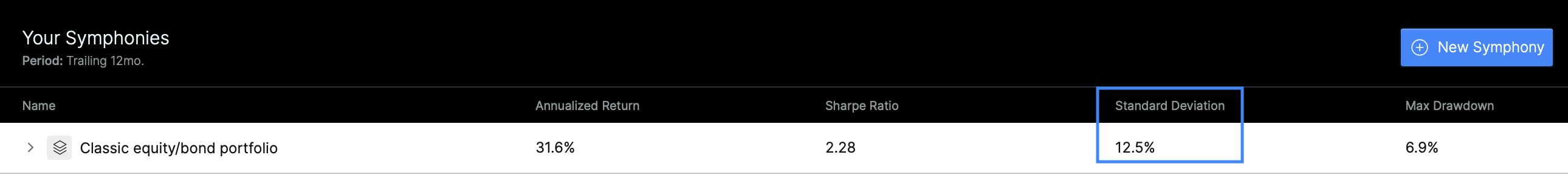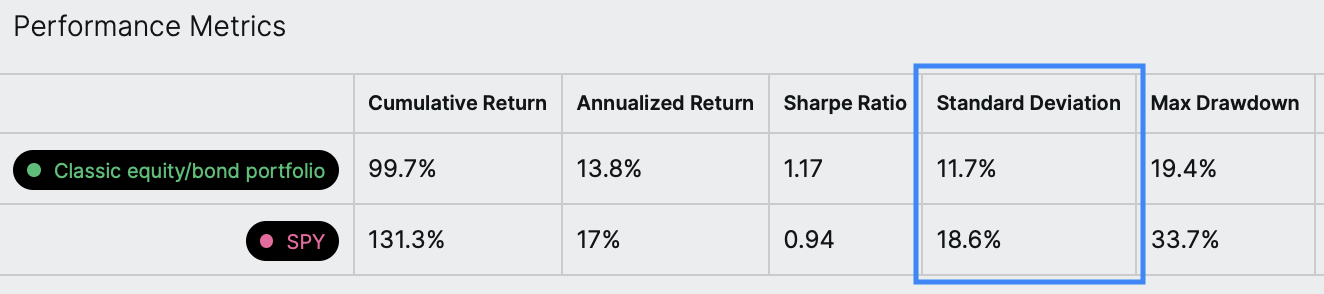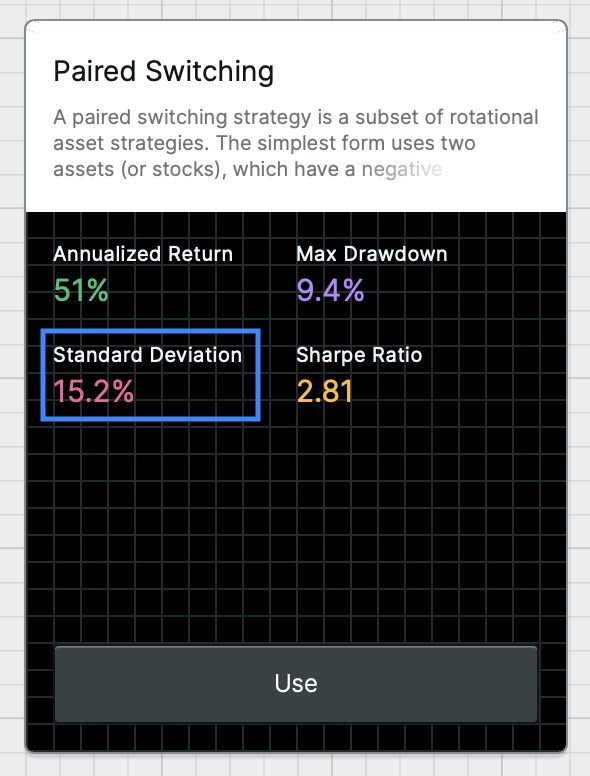# Standard deviation

What is the standard deviation?

Standard deviation is a measure of volatility, or risk, of an investment. Composer uses an annualized measure of standard deviation. The annualized standard deviation of an investment is the typical amount that the investment's daily returns vary from the mean of the returns over a selected time period, standardized to a period of a year.

When an investment's returns vary widely (i.e., when the investment is volatile), the standard deviation is high.

What is the formula?Where will you see the standard deviation in Composer?

This metric appears in a few places in Composer, including:

• In your library of symphonies• In the results of a symphony backtest• In the overview of a symphony templateWhat is the step-by-step calculation?

Let's go through the calculation of the standard deviation step-by-step for a symphony backtest. Here's how we do it:

• Compute each day’s percent return over the backtesting period
• Compute the standard deviation of those daily returns (compute the arithmetic mean of the returns, find each return’s deviation from the mean, square each deviation, calculate the sum of squares, divide the sum of squares by the number of observations to find the variance, take the square root of the variance)
• Annualize that value by multiplying by the square root of 252, the typical number of trading days in a year.